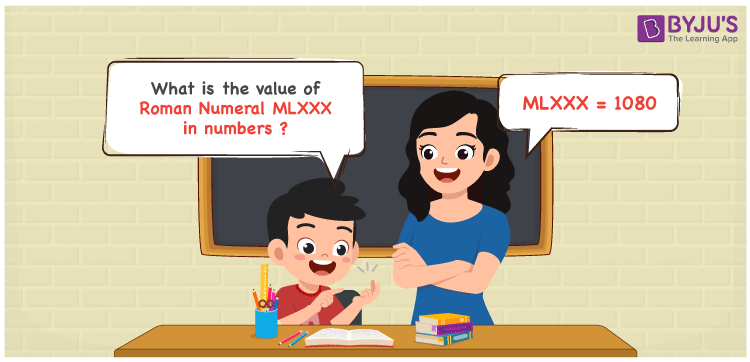Checkout JEE MAINS 2022 Question Paper Analysis : Checkout JEE MAINS 2022 Question Paper Analysis :

# MLXXX Roman Numerals

MLXXX Roman Numerals is 1080. Students can obtain a perfect understanding of the conversion of roman numerals MLXXX to numbers in this article. By reading this article on a regular basis, most of the questions in the final exams as well as various competitive exams can be answered with ease. This Roman numerals article is absolutely free of cost for the students who aim to score good marks in their exams. Therefore, MLXXX can be indicated as 1080 in numbers.

 Number Roman Numeral 1080 MLXXX## How to Write MLXXX Roman Numerals in Numbers?

Convert MLXXX to 1070 in numbers by going through the steps given in this section.

MLXXX = M + L + X + X + X

MLXXX = 1000 + 50 + 10 + 10 + 10

MLXXX = 1080

## Video Lesson on Roman Numerals## Frequently Asked Questions on MLXXX Roman Numerals

### Why is MLXXX the roman numerals of 1080?

In roman numerals

1000 is written as M

80 is written as LXXX

1080 = M + LXXX = MLXXX

### Find 1100 – 20.

We know that

1100 – 20 = 1080

Hence, 1100 + 20 is 1080 which is written as MLXXX.

### Determine the remainder we get when MLXXX is divided by VII.

We know that

MLXXX = 1080

VII = 7

So the remainder we get when MLXXX is divided by VII is II.